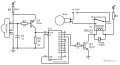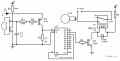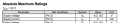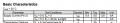#### Youssef Lakhlifi

Joined Mar 13, 2018
3
same probleme with me ,i put HCF4017BE instead CD4017 but dosn t work,View attachment 148207

#### ericgibbs

Joined Jan 29, 2010
13,626
hi Youssef,
Welcome to AAC
E

#### shortbus

Joined Sep 30, 2009
8,808
same probleme with me ,i put HCF4017BE instead CD4017 but dosn t work
You do know they are the same chip, just from different companies. If neither works in the circuit, the problem is probably the circuit.

#### MisterBill2

Joined Jan 23, 2018
9,267
You do know they are the same chip, just from different companies. If neither works in the circuit, the problem is probably the circuit.
Shortbus is correct. Unless you have a defective replacement IC, or are running it at a very high frequency. OR, verify that the reset line is low and the clock inhibit line is also low. In addition, I have seen ICs get plugged in with a pin folded under, which will cause strange problems.

#### dl324

Joined Mar 30, 2015
13,119
Some '4017 have a schmitt trigger input on clock, some don't. In addition to a schematic, we need to know the manufacturer for each of the parts.

#### Youssef Lakhlifi

Joined Mar 13, 2018
3

#### OBW0549

Joined Mar 2, 2015
3,566
Your diagram shows Q1 as a BC557, which is a PNP transistor. Q1 needs to be an NPN or the circuit will not work. Also, the data sheet for the TSOP1738 clearly shows the maximum allowable supply voltage for the part is 6 volts; using a 9 volt supply, you've quite possibly destroyed it.

#### ScottWang

Joined Aug 23, 2012
7,065
1. Q1 - use BBC556 or 2N3906 and reverse E,C, E connected to positive.
2. TSOP1738 and the E(BC556) of Q1 in series with a 820 Ω resistor(R5) to 9V.
3. If the relay is use 6V and the Vcc is 9V then the R4=(9V-6V)/I_relay=X, the X values can be a little lower than the calculation values.
4. R1 increase to 22K, R2 decrease to 22K~47K.
5. The new circuit for your reference as below.#### OBW0549

Joined Mar 2, 2015
3,566
@ScottWang, that circuit still leaves the TSOP1738 with excessive supply voltage: when its output goes HI, the voltage on its +Vs terminal will follow, 0.7V above the output voltage, until it approaches the 9V input voltage.

#### ScottWang

Joined Aug 23, 2012
7,065
@ScottWang, that circuit still leaves the TSOP1738 with excessive supply voltage: when its output goes HI, the voltage on its +Vs terminal will follow, 0.7V above the output voltage, until it approaches the 9V input voltage.
Thanks.
I was calculated the current of Vs less than 5mA, so if the TSOP1738 works as current load then it will be ok, if it is works as voltage load then probably it will be as your concerned and damaged, I think many ee parts(semiconductor) are the current load, so I will treated TSOP1738 as current load.

#### OBW0549

Joined Mar 2, 2015
3,566
I was calculated the current of Vs less than 5mA, so if the TSOP1738 works as current load then it will be ok, if it is works as voltage load then probably it will be as your concerned and damaged, I think many ee parts(semiconductor) are the current load, so I will treated TSOP1738 as current load.
The TSOP1738 data sheet lists its supply current as 0.4 mA to 0.8 mA. With R5 = 820Ω, the resulting voltage drop leaves the +Vs pin with between 8.34V and 8.67V, which exceeds the part's 6V Absolute Maximum Rating for supply voltage.

If the TS really wants to use the circuit you posted, he needs to insert a 5.1V Zener diode (1N4733 or equiv.) between the TSOP1738's +Vs pin and circuit GND.

#### ScottWang

Joined Aug 23, 2012
7,065
The TSOP1738 data sheet lists its supply current as 0.4 mA to 0.8 mA. With R5 = 820Ω, the resulting voltage drop leaves the +Vs pin with between 8.34V and 8.67V, which exceeds the part's 6V Absolute Maximum Rating for supply voltage.
What your said were about the voltage load, but I'm talking about the current load, I haven't use the TSOP1738, so I can't make sure which one is right, I just according to the experienced.

If the TS really wants to use the circuit you posted, he needs to insert a 5.1V Zener diode (1N4733 or equiv.) between the TSOP1738's +Vs pin and circuit GND.
If use the other IC or device probably that is a easy way, but the problem here is the current limited to too less, so if use zener diode maybe the current will be too much, and that is what the datasheet mentioned.

#### OBW0549

Joined Mar 2, 2015
3,566
If use the other IC or device probably that is a easy way, but the problem here is the current limited to too less, so if use zener diode maybe the current will be too much, and that is what the datasheet mentioned.
I'm sorry, but that doesn't make any sense to me. Connecting a Zener diode across the TSOP1738's +Vs and GND pins simply clamps its supply voltage to a maximum of Vz; it does not-- and can not-- cause the supply current drawn by the TSOP1738 to increase.

Also, I'm not sure what you mean by "voltage load" or "current load." Are you distinguishing between supplying the part with a constant voltage across +Vs and GND, versus supplying it with a constant current injected into the +Vs pin? The TSOP1738 is powered by a voltage across +Vs and GND, like nearly all other ICs in common usage.

#### MisterBill2

Joined Jan 23, 2018
9,267
I'm sorry, but that doesn't make any sense to me. Connecting a Zener diode across the TSOP1738's +Vs and GND pins simply clamps its supply voltage to a maximum of Vz; it does not-- and can not-- cause the supply current drawn by the TSOP1738 to increase.

Also, I'm not sure what you mean by "voltage load" or "current load." Are you distinguishing between supplying the part with a constant voltage across +Vs and GND, versus supplying it with a constant current injected into the +Vs pin? The TSOP1738 is powered by a voltage across +Vs and GND, like nearly all other ICs in common usage.
What I see is a very real possibility that the voltage at the clock pin never goes low enough to count as a zero. Putting a high impedance meter on it and checking is a good start. Then I am wondering just what the purpose of the circuit is, since it seems that the relay will alternate states on consecutive pulses. That can be done with a CD4013 flipflop just as easily and have an extra one for free. Whit the original circuit and the 100K pull down resistor I doubt that the counter ever saw an input state change, so it would never do anything. I would suggest an input pull-down resistor of 10K ohms at most. Otherwise, just a bit of transistor leakage and the state never goes to zero.

#### ScottWang

Joined Aug 23, 2012
7,065
I'm sorry, but that doesn't make any sense to me. Connecting a Zener diode across the TSOP1738's +Vs and GND pins simply clamps its supply voltage to a maximum of Vz; it does not-- and can not-- cause the supply current drawn by the TSOP1738 to increase.
As your suggested would be adds one more protection and I re-calculated the values of resistors and redrew the circuit below.

The Is of TSOP1738 >= 5mA, Iz >= 5mA, Iout <=5mA.
R5 = (9V-5.1V)/(Is + Iz + Iout+1mA) = 4.9V/(5mA+5mA+5mA +1 mA) = 306Ω (choose 300Ω) ------ choose more than 15 mA, so plus 1mA.
R1+R2 =(5.1V-Q1_Vbe-Vout_low)
= (5.1V-0.7V-0.2V)/5mA
= 840Ω (if use 910Ω to get 4.6 mA (Iout) maybe also can be works ok.)Also, I'm not sure what you mean by "voltage load" or "current load." Are you distinguishing between supplying the part with a constant voltage across +Vs and GND, versus supplying it with a constant current injected into the +Vs pin? The TSOP1738 is powered by a voltage across +Vs and GND, like nearly all other ICs in common usage.
What I said that the current load was to described if use the resistor to limits the current less than the rating current and then the load will be no harm, and the voltage load was to described when the voltage exceed the rating voltage then the load could be damaged.

#### OBW0549

Joined Mar 2, 2015
3,566
The Is of TSOP1738 >= 5mA
Where in the world did you get that idea? If you got it from the data sheet, here:you need to understand that that 5 mA is a safety rating; it says, in effect, "no matter how you are using this device, do not EVER cause more than 5 mA to be forced into the +Vs supply pin."

That number does NOT tell you the supply current the part will draw; instead, the supply current required by the part is given in the Basic Characteristics section, here:The TSOP1738 will draw 0.4 mA minimum, 0.6 mA typical, 0.8 mA maximum. That's the amount of current the TSOP1738 will draw at a supply voltage of 5 volts. Not 5 mA.

•ScottWang

#### ScottWang

Joined Aug 23, 2012
7,065
Where in the world did you get that idea? If you got it from the data sheet, here:

View attachment 148406

you need to understand that that 5 mA is a safety rating; it says, in effect, "no matter how you are using this device, do not EVER cause more than 5 mA to be forced into the +Vs supply pin."
Thank you.
I know what you mean, when I saw the V/I militation values, I still didn't realised that the TSOP1738 is a V/I limits component like as a LED, and the idea came out, I thought that it is a normal IC, and we can provide a unlimited current to a normal IC, so the idea covered the values of specification, that was why I used ">" symbol.

That number does NOT tell you the supply current the part will draw; instead, the supply current required by the part is given in the Basic Characteristics section, here:

View attachment 148407

The TSOP1738 will draw 0.4 mA minimum, 0.6 mA typical, 0.8 mA maximum. That's the amount of current the TSOP1738 will draw at a supply voltage of 5 volts. Not 5 mA.
Before I draw the circuit, I would like to know what is your best solution to match the voltage and current limitation for this application.

#### OBW0549

Joined Mar 2, 2015
3,566
Before I draw the circuit, I would like to know what is your best solution to match the voltage and current limitation for this application.
I would do exactly what I suggested in post #11: use your circuit of post #8, but add a 5.1V Zener diode across the +Vs and GND pins of the TSOP1738 to limit its supply to a safe value.

That is all that is necessary.

#### ScottWang

Joined Aug 23, 2012
7,065
I would do exactly what I suggested in post #11: use your circuit of post #8, but add a 5.1V Zener diode across the +Vs and GND pins of the TSOP1738 to limit its supply to a safe value.

That is all that is necessary.
I would like to see the values of resistors and the current for Vs, Out, and zener diode.

#### OBW0549

Joined Mar 2, 2015
3,566
I would like to see the values of resistors and the current for Vs, Out, and zener diode.
Leave the component values exactly as you had them in post #8.

With the Zener diode added, supply voltage for the TSOP1738 is 5.1 volts.
The current through R5 is (9V - 5.1V) / 820Ω = 4.75 mA.
The current drawn by the TSOP1738 is 0.8 mA maximum.
With the TSOP1738 output low, the current drawn by R1 and R2 together is approx. 0.5 mA.
The minimum current available for the Zener diode, therefore, is (4.75 mA - 0.8 mA - 0.5 mA) = 3.45 mA, which is quite sufficient.